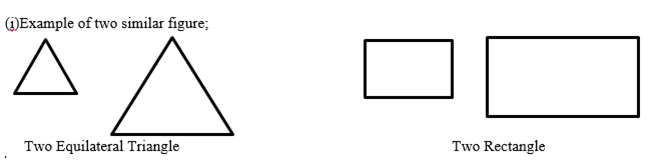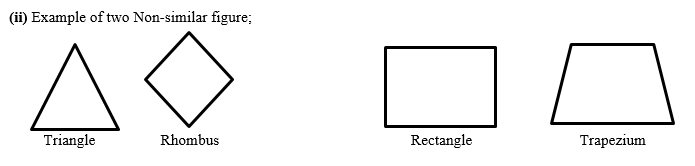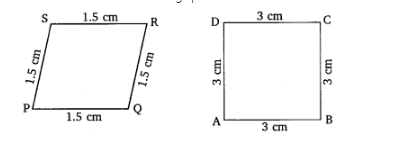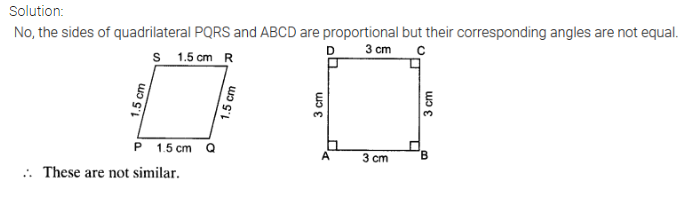• +91 9971497814
• info@interviewmaterial.com

# Triangles EX-6.1 Interview Questions Answers

### Related Subjects

Question 1 : Fill in the blanks using correct word given in the brackets:-

Answer 1 : (i) All circles are __________. (congruent, similar)

(ii) All squares are __________. (similar, congruent)
(iii) All __________ triangles are similar. (isosceles, equilateral)
(iv) Two polygons of the same number of sides are similar, if (a) their corresponding angles are __________ and (b) their corresponding sides are __________. (equal, proportional)

(i) All circles are __________. (congruent, similar)

(ii) All squares are __________. (similar, congruent)

(iii) All __________ triangles are similar. (isosceles, equilateral)

(iv) Two polygons of the same number of sides are similar, if (a) their corresponding angles are __________ and (b) their corresponding sides are __________. (equal, proportional)
(b) Proportional

Question 2 :
Give two different examples of pair of
(i) Similar figures
(ii) Non-similar figures

Answer 2 :Question 3 : State whether the following quadrilaterals are similar or not.

Answer 3 :Todays Deals### Triangles EX-6.1 Contributorskrishan

Name:
Email:

# Latest News# 9000 interview questions in different categories